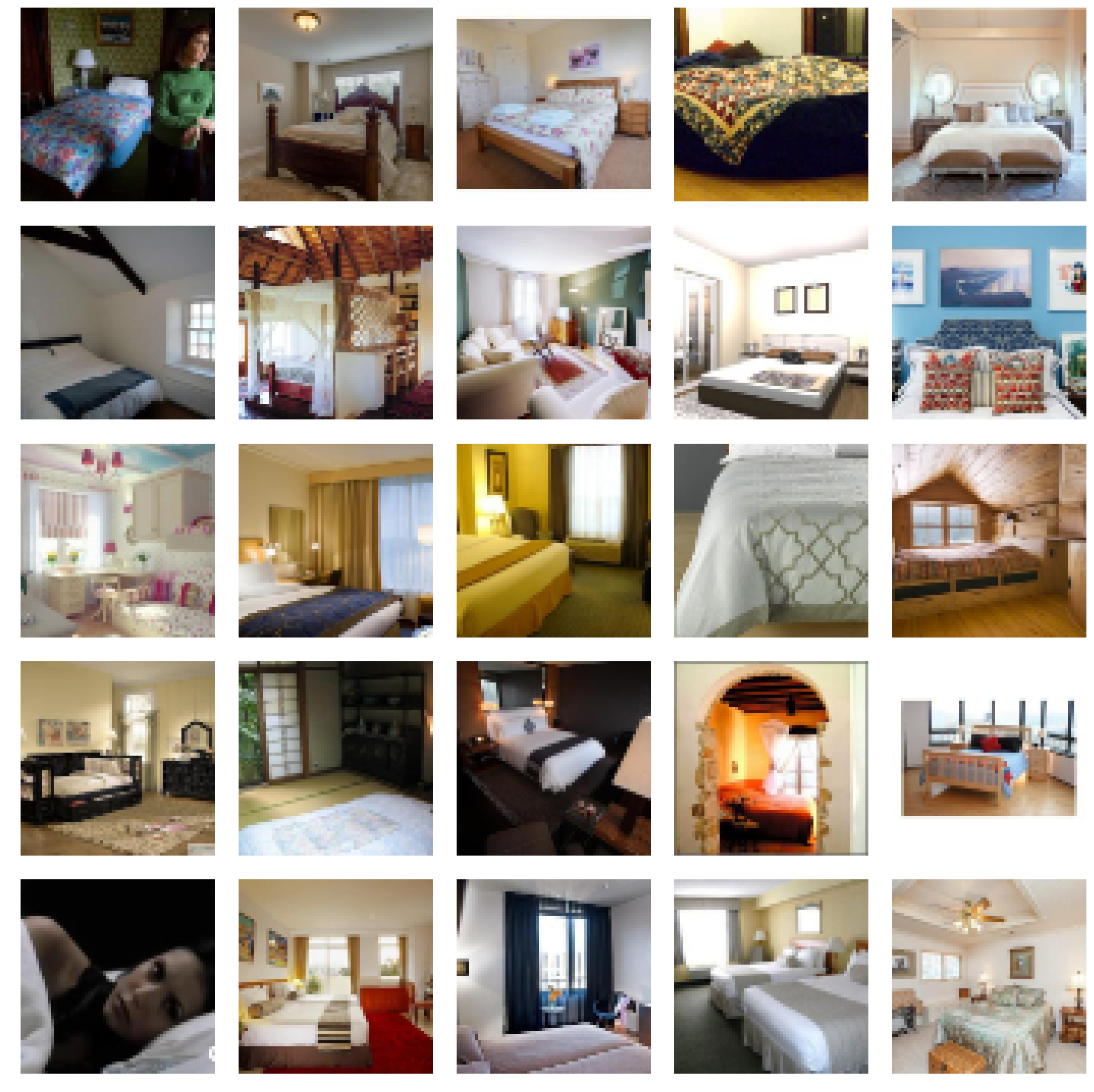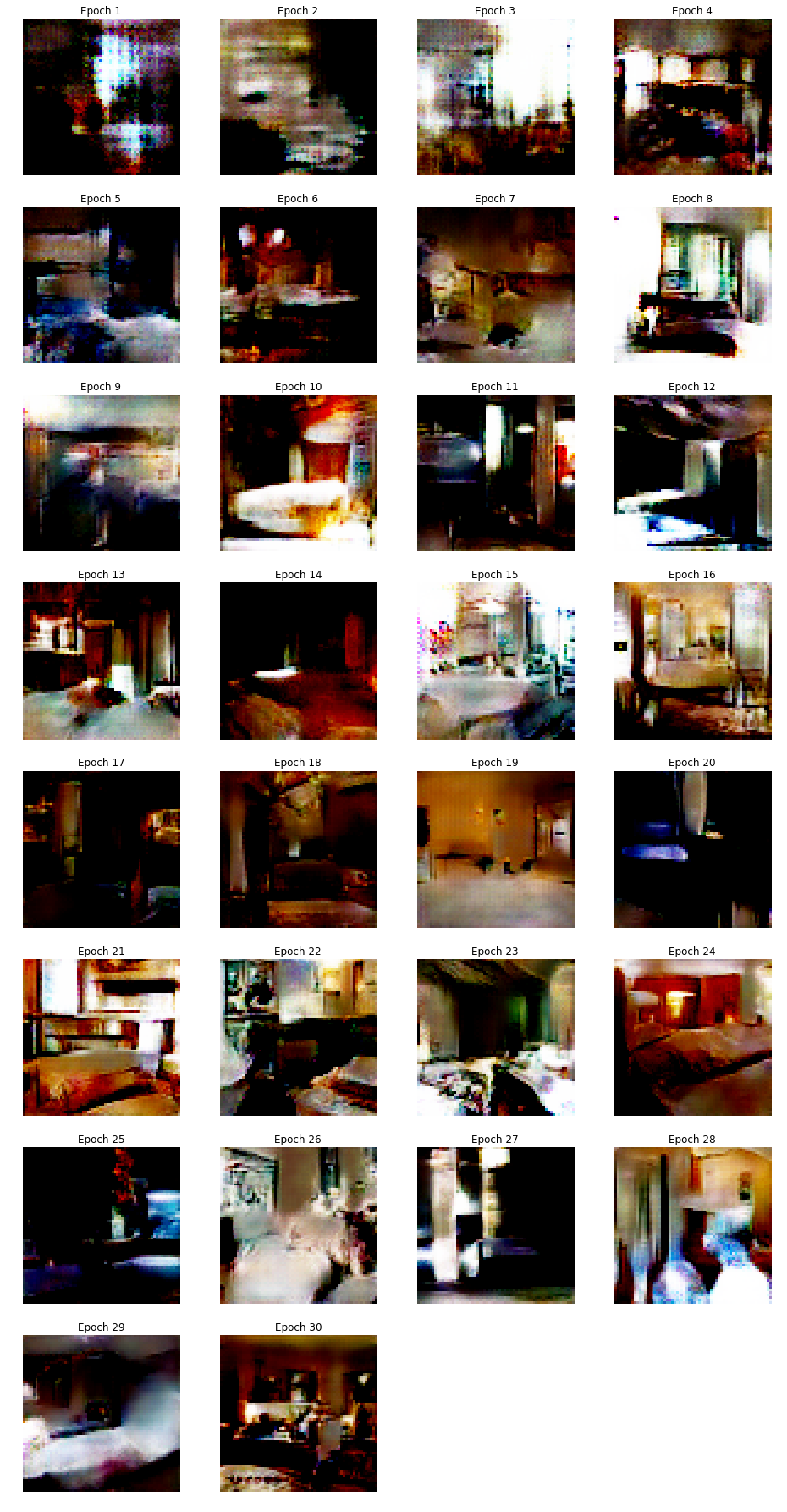In [ ]:
%reload_ext autoreload
%matplotlib inline

In [ ]:
from fastai.vision import *
from fastai.vision.gan import *


## LSun bedroom data¶

For this lesson, we'll be using the bedrooms from the LSUN dataset. The full dataset is a bit too large so we'll use a sample from kaggle.

In [ ]:
path = untar_data(URLs.LSUN_BEDROOMS)


We then grab all the images in the folder with the data block API. We don't create a validation set here for reasons we'll explain later. It consists of random noise of size 100 by default (can be changed below) as inputs and the images of bedrooms as targets. That's why we do tfm_y=True in the transforms, then apply the normalization to the ys and not the xs.

In [ ]:
def get_data(bs, size):
return (GANItemList.from_folder(path, noise_sz=100)
.split_none()
.label_from_func(noop)
.transform(tfms=[[crop_pad(size=size, row_pct=(0,1), col_pct=(0,1))], []], size=size, tfm_y=True)
.databunch(bs=bs)
.normalize(stats = [torch.tensor([0.5,0.5,0.5]), torch.tensor([0.5,0.5,0.5])], do_x=False, do_y=True))


We'll begin with a small side and use gradual resizing.

In [ ]:
data = get_data(128, 64)

In [ ]:
data.show_batch(rows=5)## Models¶

GAN stands for Generative Adversarial Nets and were invented by Ian Goodfellow. The concept is that we will train two models at the same time: a generator and a critic. The generator will try to make new images similar to the ones in our dataset, and the critic will try to classify real images from the ones the generator does. The generator returns images, the critic a single number (usually 0. for fake images and 1. for real ones).

We train them against each other in the sense that at each step (more or less), we:

1. Freeze the generator and train the critic for one step by:
• getting one batch of true images (let's call that real)
• generating one batch of fake images (let's call that fake)
• have the critic evaluate each batch and compute a loss function from that; the important part is that it rewards positively the detection of real images and penalizes the fake ones
• update the weights of the critic with the gradients of this loss
1. Freeze the critic and train the generator for one step by:
• generating one batch of fake images
• evaluate the critic on it
• return a loss that rewards posisitivly the critic thinking those are real images; the important part is that it rewards positively the detection of real images and penalizes the fake ones
• update the weights of the generator with the gradients of this loss

Here, we'll use the Wassertein GAN.

We create a generator and a critic that we pass to gan_learner. The noise_size is the size of the random vector from which our generator creates images.

In [ ]:
generator = basic_generator(in_size=64, n_channels=3, n_extra_layers=1)
critic    = basic_critic   (in_size=64, n_channels=3, n_extra_layers=1)

In [ ]:
learn = GANLearner.wgan(data, generator, critic, switch_eval=False,
opt_func = partial(optim.Adam, betas = (0.,0.99)), wd=0.)

In [ ]:
learn.fit(30,2e-4)

Total time: 1:54:23

epoch train_loss gen_loss disc_loss
1 -0.842719 0.542895 -1.086206
2 -0.799776 0.539448 -1.067940
3 -0.738768 0.538581 -1.015152
4 -0.718174 0.484403 -0.943485
5 -0.570070 0.428915 -0.777247
6 -0.545130 0.413026 -0.749381
7 -0.541453 0.389443 -0.719322
8 -0.469548 0.356602 -0.642670
9 -0.434924 0.329100 -0.598782
10 -0.416448 0.301526 -0.558442
11 -0.389224 0.292355 -0.532662
12 -0.361795 0.266539 -0.494872
13 -0.363674 0.245725 -0.475951
14 -0.318343 0.227446 -0.432148
15 -0.309221 0.203628 -0.417945
16 -0.300667 0.213194 -0.401034
17 -0.282622 0.187128 -0.381643
18 -0.283902 0.156653 -0.374541
19 -0.267852 0.159612 -0.346919
20 -0.257258 0.163018 -0.344198
21 -0.242090 0.159207 -0.323443
22 -0.255733 0.129341 -0.322228
23 -0.235854 0.143768 -0.305106
24 -0.220397 0.115682 -0.289971
25 -0.233782 0.135361 -0.294088
26 -0.202050 0.142435 -0.279994
27 -0.196104 0.119580 -0.265333
28 -0.204124 0.119595 -0.266063
29 -0.204096 0.131431 -0.264097
30 -0.183655 0.128817 -0.254156In [ ]:
learn.gan_trainer.switch(gen_mode=True)
learn.show_results(ds_type=DatasetType.Train, rows=16, figsize=(8,8))